Download the eSaral app and start learning from Kota's top IITians and doctors.

# NCERT Exemplar Class 9 Maths Chapter 4 - Linear Equations in Two variables - Free PDF download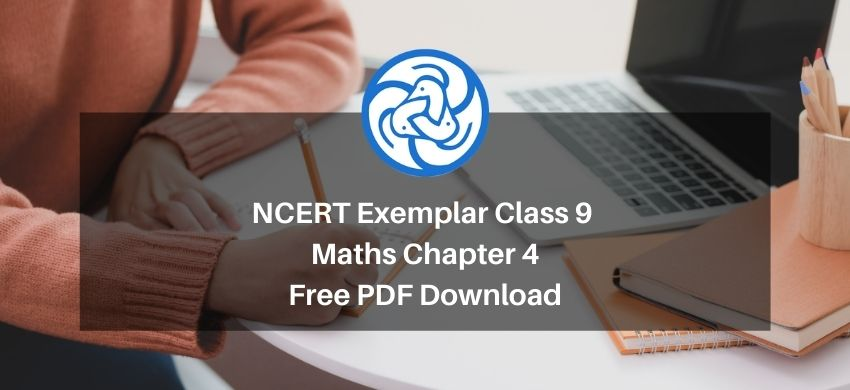Hey, are you a class 9 student? and looking for ways to download NCERT Exemplar Class 9 Maths Chapter 4 "Linear Equations in Two variables"? If yes. Then read this post till the end.

If you want to score high in your Class 9 Maths exam then it is very important for you to understand and learn the topics of Class 9 Maths thoroughly.

So, without wasting more time let’s start.

### Download The PDF of NCERT Exemplars Class 9 Maths Chapter 4 "Linear Equations in Two variables"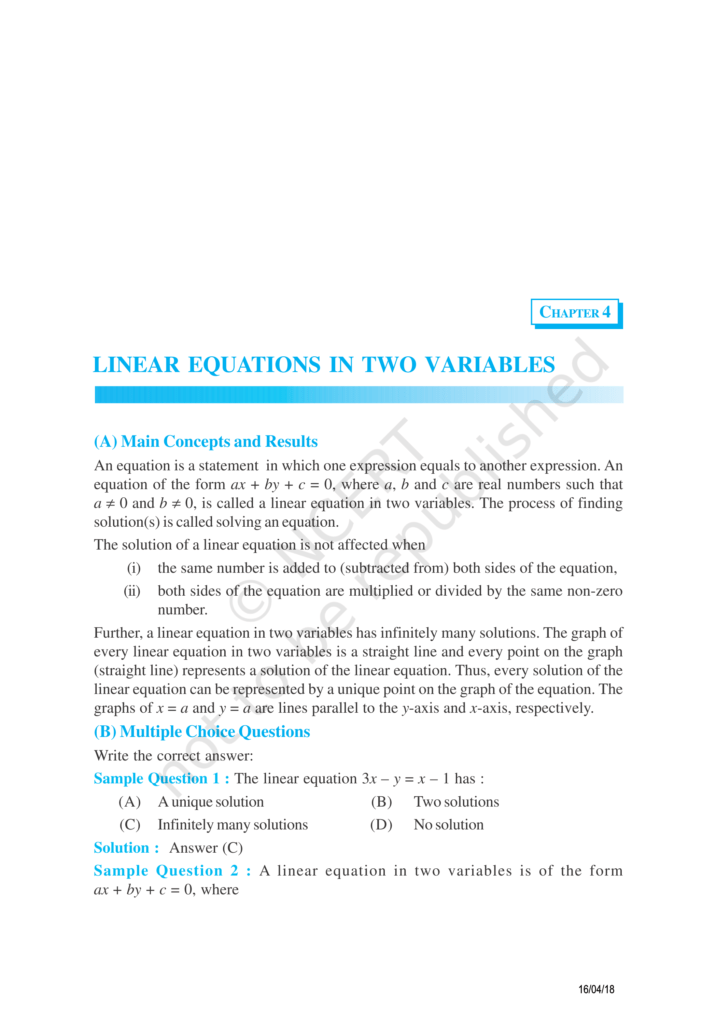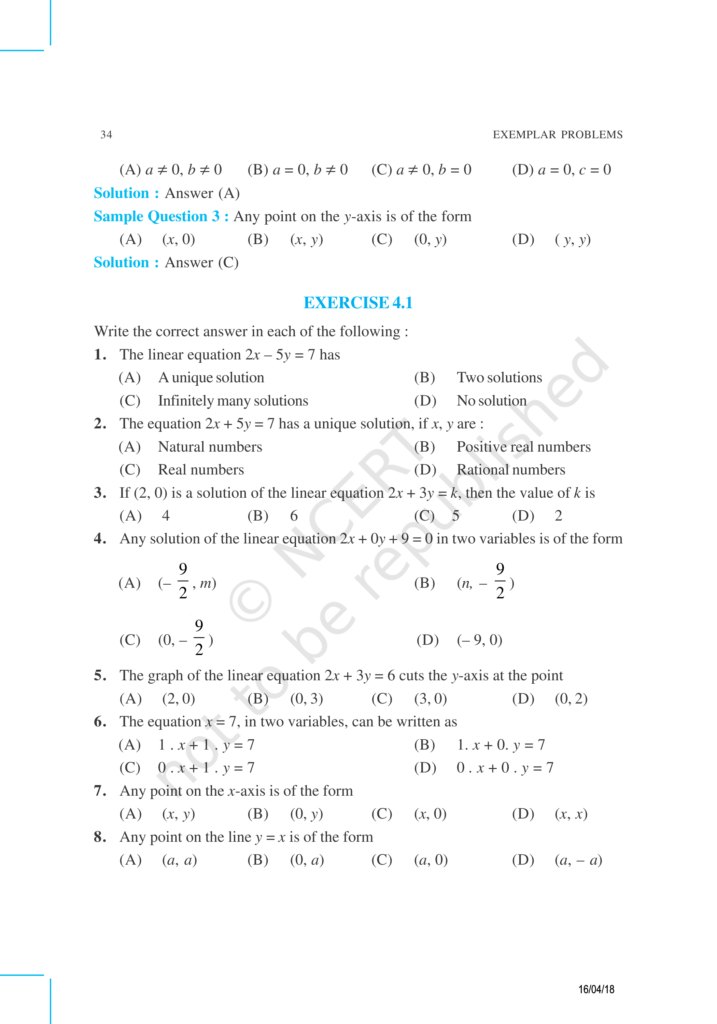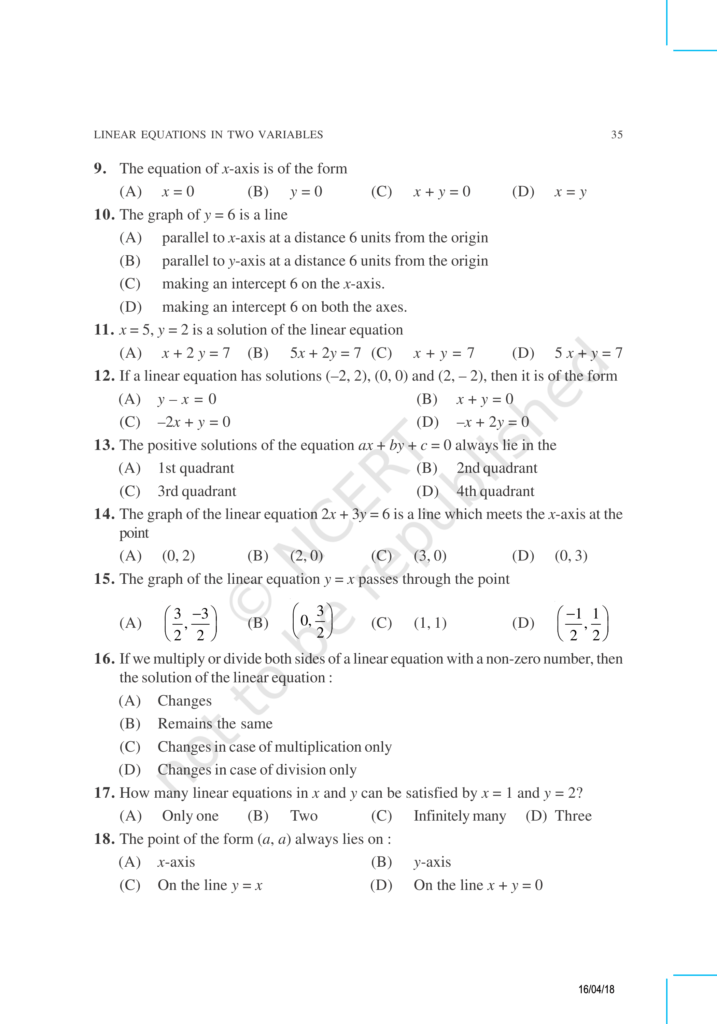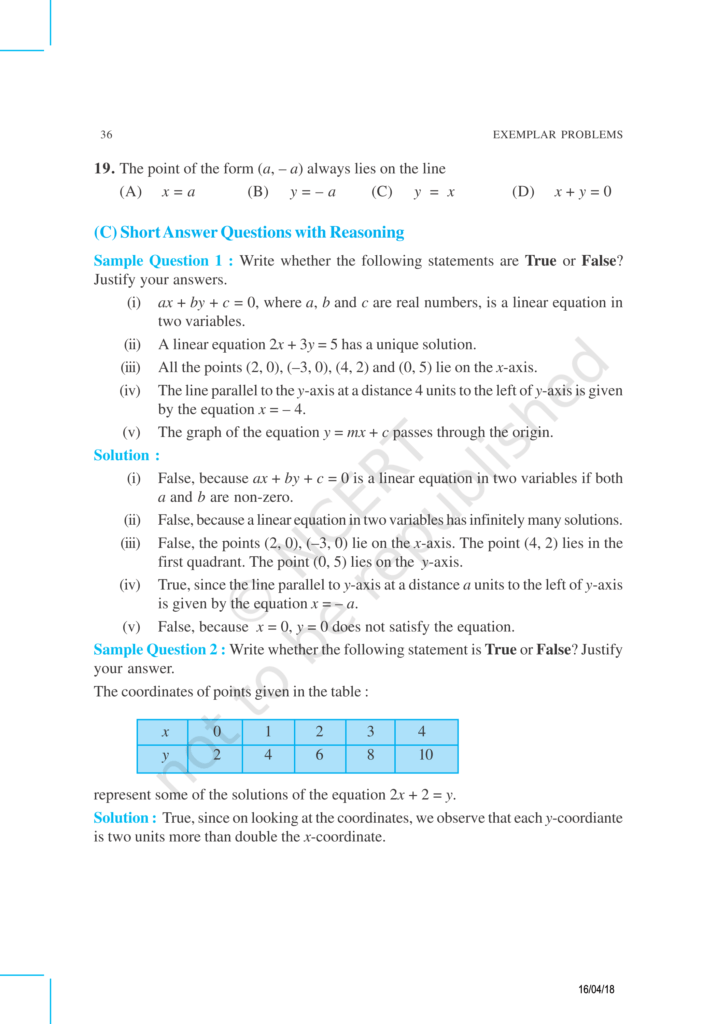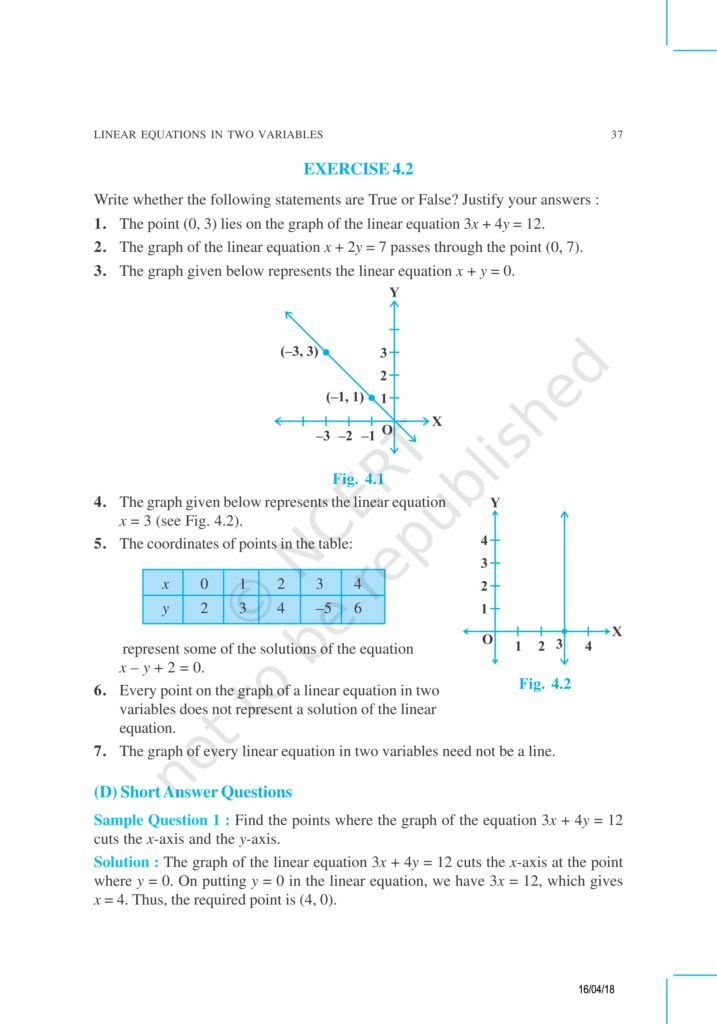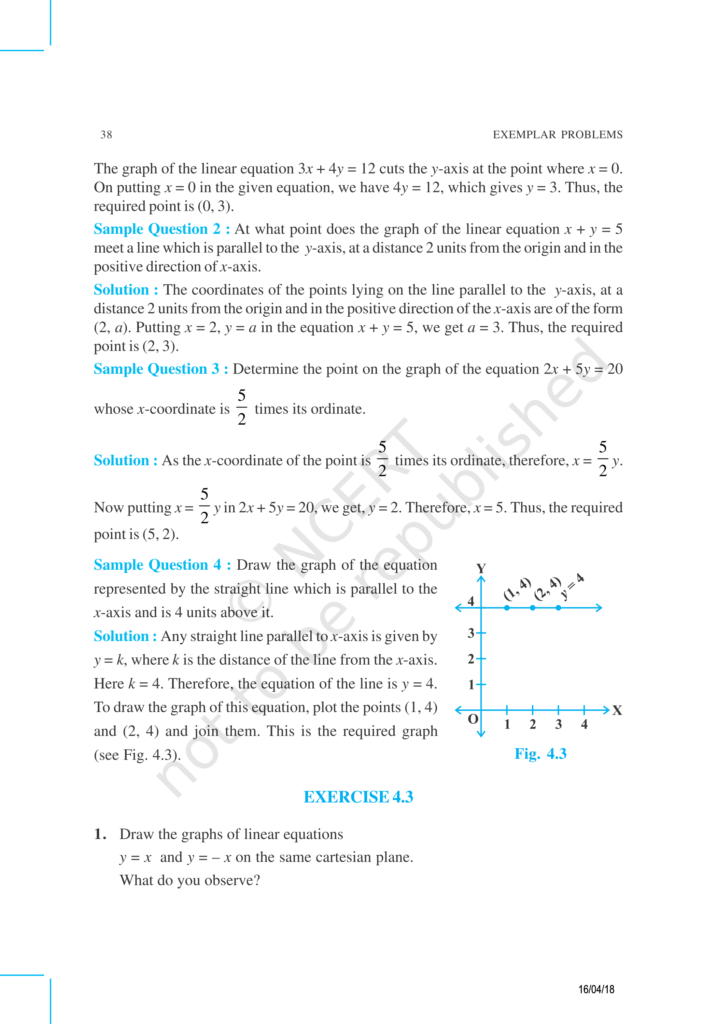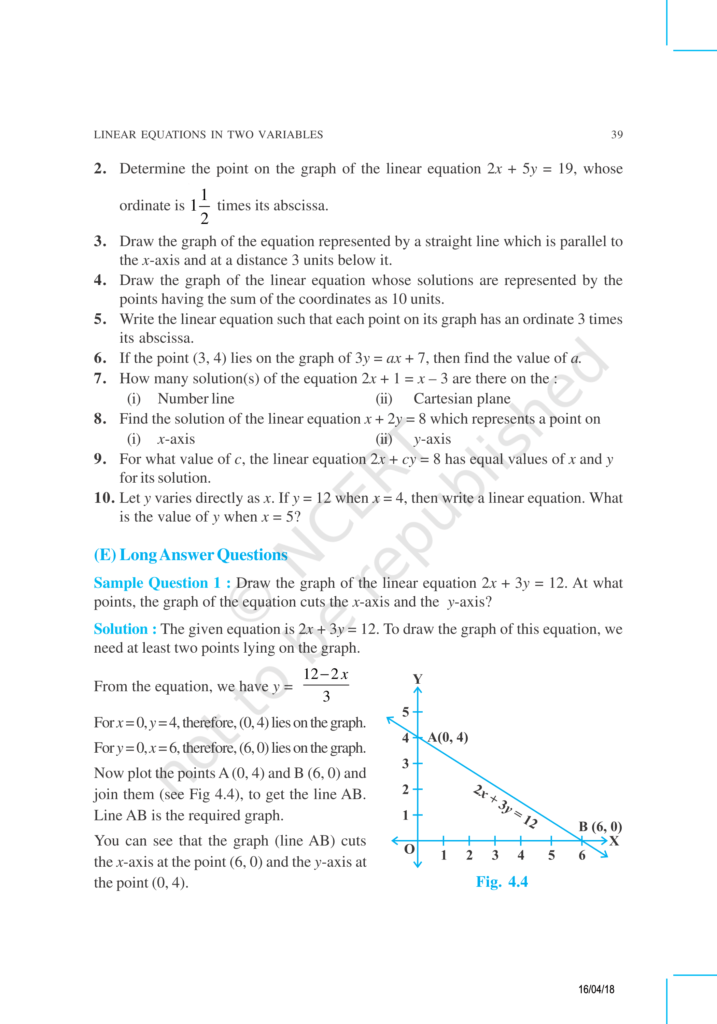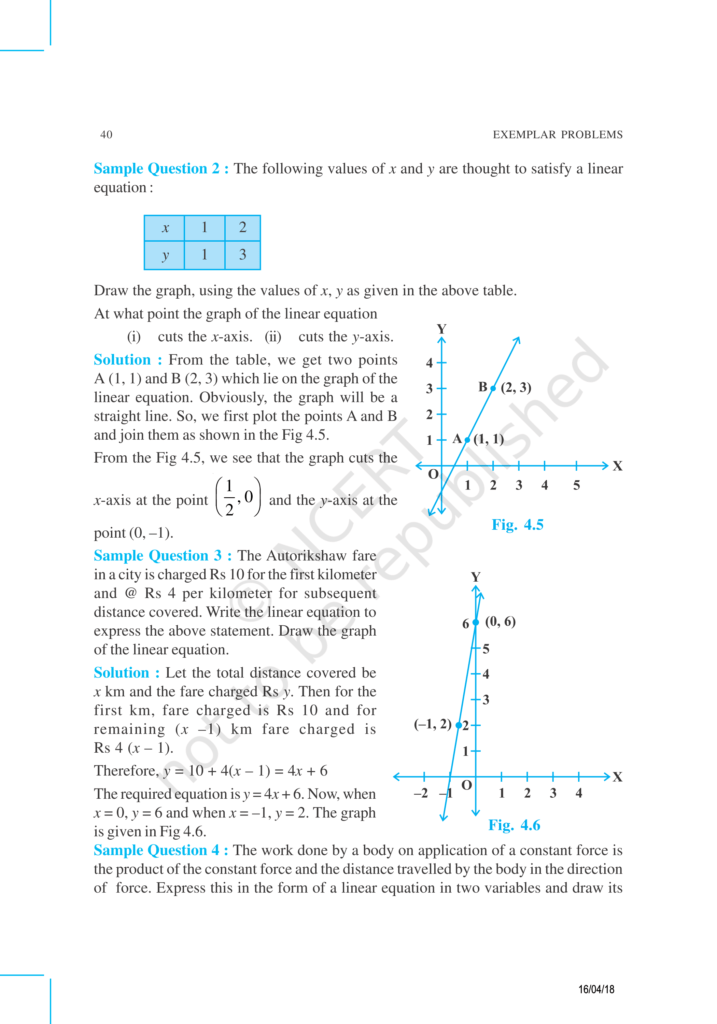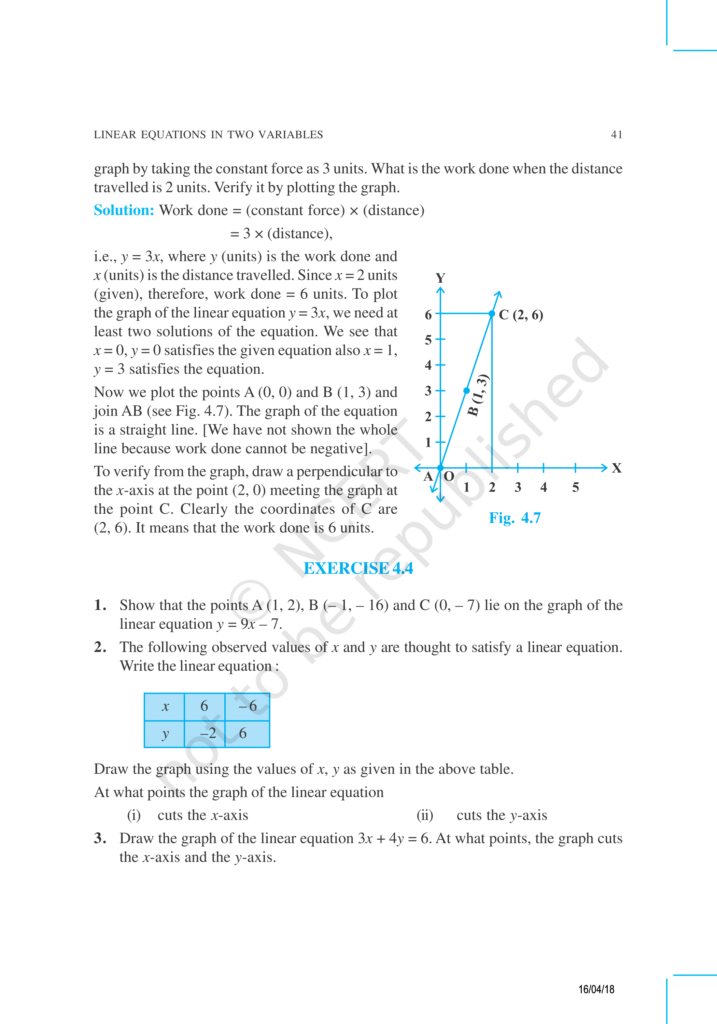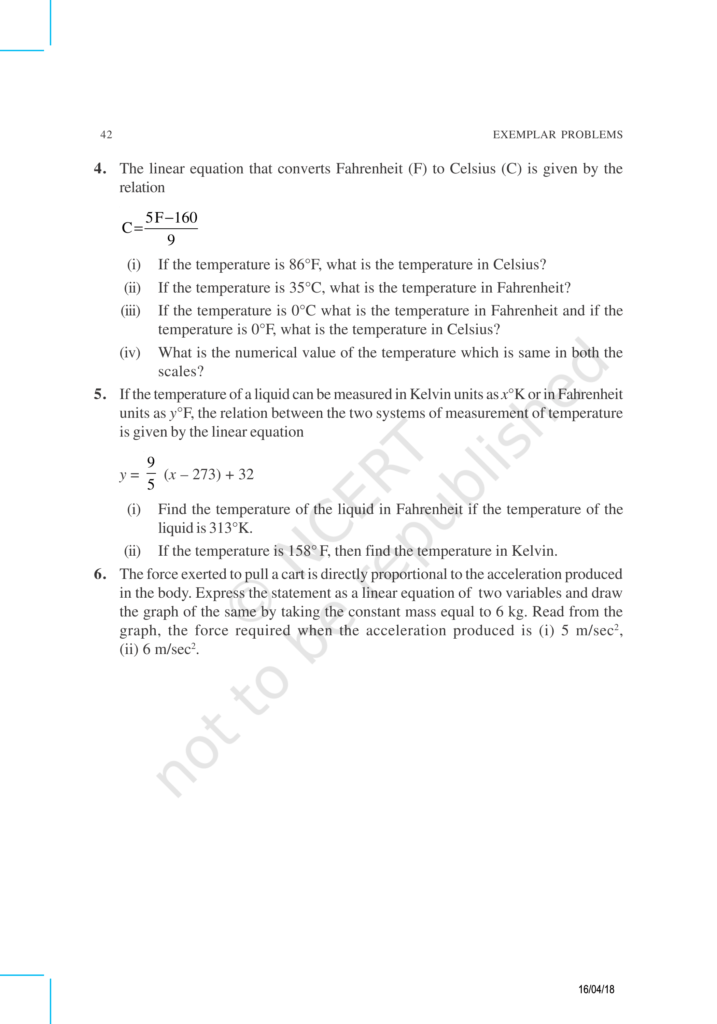If you have any Confusion related to NCERT Exemplar Class 9 Maths Chapter 4 then feel free to ask in the comments section down below.

To watch Free Learning Videos on Class 9 Maths by Kota’s top Faculties Install the eSaral App